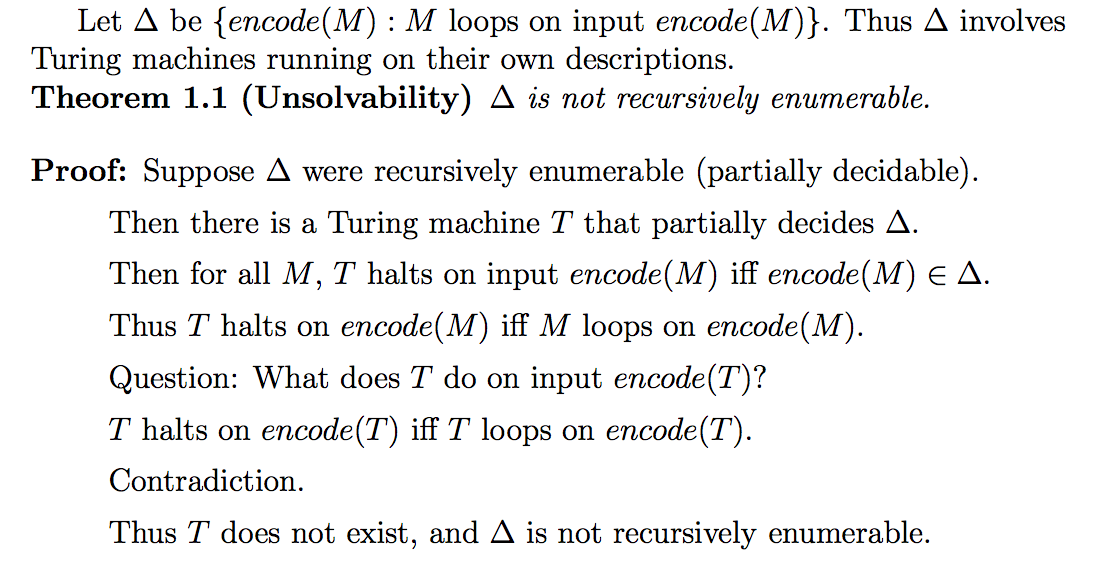```Things to do to prepare for final:

Understand deterministic vs nondeterministic in context free languages.

Why is language of same number of a’s b’s and c’s be nondeterministic context free?

Know that when you have (i,j) such that T_i halts on j, this as a language is partially decidable.

Nothing can add to the power of a turing machine.

Review the pumping lemma for context free a little it more.

REVIEW HOW TO PROOF HALTING PROBLEM THING.

What does the dollar sign mean? Just assume it has the same properties

What is a reduction? An algorithm for transforming one problem into another problem.

If L(M) = L and M is nondeterministic then M has at least n states IS FALSE. WHY?

Remember: Does/doesn’t contain substring will very likely be regular language.

Remember: DON’T ASSUME DETERMINISTIC.

Remember:
Regular are closed under: L1 U L2 (union), L1L2 (concatenation), L1* (kleene star), L1 intersect L2 (intersection).
Context free is closed under: union, concatenation, klene star, union with regular, intersection with regular.

Main things:

-Review context free pumping. http://www.cs.unc.edu/~plaisted/comp455/hndout6.pdf
If L is a language and for all integers N, there is a string w ∈ L of length
greater than N such that for all ways of writing w as uvxyz with (v not equal e or
y not equal = e), there is an i such that uv^ixy^iz is not in L, then L is not context-free.

Remember: a^n b^m c^p where m neql n, or m neql p is NONDETERMINISTIC CONTEXT FREE.

T halts on input encode(M) iff encode(M) in delta
Thus T halts on encode(M) iff M {{loops on encode(M)}}.
↓
{{loops on encode(T)}}

-Proof problem at the end.
Let L be the set of all Turing machines that do not halt on the input of themselves. Show there is no Turing machine that halts on i, if i is in L.

Essentially, show that there is no Turing machine that halts
Quick note: “M” is the Turing Machine M but written as a string, so M equiv to T_i.
Apparently this proof problem is equivalent to asking: show the set “M” such that M does not halt on “M” is not partially decidable.

Approach: try making this a reduction of the halting problem.

Here’s the proof:￼￼

Jist:
For all M, T halts on input encode(M) iff encode(M) in Delta.
Thus T halts on encode(M) iff M loops on encode(M).
T halts on encode(T) iff T loops on encode(T).